Home Practice
For learners and parents For teachers and schools
Textbooks
Full catalogue
Pricing SupportLog in

We think you are located in United States. Is this correct?

# 11.2 Ohm's Law

## 11.2 Ohm's Law (ESBQ6)

temp text

Three quantities which are fundamental to electric circuits are current, voltage (potential difference) and resistance. To recap:

1. Electrical current, $$I$$, is defined as the rate of flow of charge through a circuit.

2. Potential difference or voltage, $$V$$, is the amount of energy per unit charge needed to move that charge between two points in a circuit.

3. Resistance, $$R$$, is a measure of how `hard' it is to push current through a circuit element.

We will now look at how these three quantities are related to each other in electric circuits.

An important relationship between the current, voltage and resistance in a circuit was discovered by Georg Simon Ohm and it is called Ohm's Law.

Ohm's Law

The amount of electric current through a metal conductor, at a constant temperature, in a circuit is proportional to the voltage across the conductor and can be described by

$$I = \frac{V}{R}$$

where $$I$$ is the current through the conductor, $$V$$ is the voltage across the conductor and $$R$$ is the resistance of the conductor. In other words, at constant temperature, the resistance of the conductor is constant, independent of the voltage applied across it or current passed through it.

Ohm's Law tells us that if a conductor is at a constant temperature, the current flowing through the conductor is directly proportional to the voltage across it. This means that if we plot voltage on the x-axis of a graph and current on the y-axis of the graph, we will get a straight-line.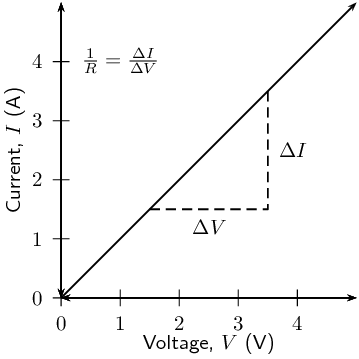The gradient of the straight-line graph is related to the resistance of the conductor as $\frac{I}{V} = \frac{1}{R}$ This can be rearranged in terms of the constant resistance as: $R = \frac{V}{I}$

temp text

## Ohm's Law

### Aim

To determine the relationship between the current going through a resistor and the potential difference (voltage) across the same resistor.

### Apparatus

4 cells, 4 resistors, an ammeter, a voltmeter, connecting wires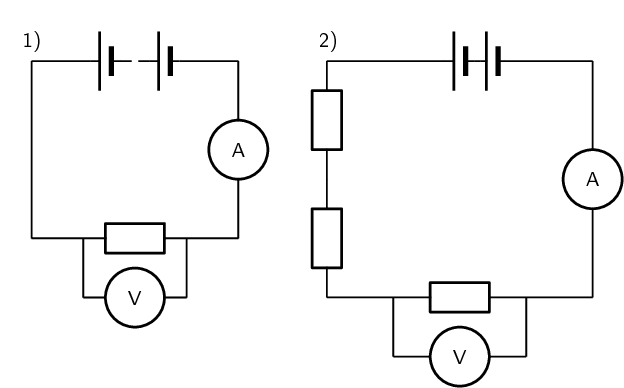### Method

This experiment has two parts. In the first part we will vary the applied voltage across the resistor and measure the resulting current through the circuit. In the second part we will vary the current in the circuit and measure the resulting voltage across the resistor. After obtaining both sets of measurements, we will examine the relationship between the current and the voltage across the resistor.

1. Varying the voltage:

1. Set up the circuit according to circuit diagram 1), starting with just one cell.

2. Draw the following table in your lab book.

 Number of cells Voltage, V ($$\text{V}$$) Current, I ($$\text{A}$$) $$\text{1}$$ $$\text{2}$$ $$\text{3}$$ $$\text{4}$$
3. Get your teacher to check the circuit before turning the power on.

4. Measure the voltage across the resistor using the voltmeter, and the current in the circuit using the ammeter.

5. Add one more $$\text{1,5}$$ $$\text{V}$$ cell to the circuit and repeat your measurements.

6. Repeat until you have four cells and you have completed your table.

2. Varying the current:

1. Set up the circuit according to circuit diagram 2), starting with only 1 resistor in the circuit.

2. Draw the following table in your lab book.

 Voltage, V ($$\text{V}$$) Current, I ($$\text{A}$$)
3. Get your teacher to check your circuit before turning the power on.

4. Measure the current and measure the voltage across the single resistor.

5. Now add another resistor in series in the circuit and measure the current and the voltage across only the original resistor again. Continue adding resistors until you have four in series, but remember to only measure the voltage across the original resistor each time. Enter the values you measure into the table.

### Analysis and results

1. Using the data you recorded in the first table, draw a graph of current versus voltage. Since the voltage is the variable which we are directly varying, it is the independent variable and will be plotted on the $$x$$-axis. The current is the dependent variable and must be plotted on the $$y$$-axis.

2. Using the data you recorded in the second table, draw a graph of voltage vs. current. In this case the independent variable is the current which must be plotted on the $$x$$-axis, and the voltage is the dependent variable and must be plotted on the $$y$$-axis.

### Conclusions

1. Examine the graph you made from the first table. What happens to the current through the resistor when the voltage across it is increased? i.e. Does it increase or decrease?

2. Examine the graph you made from the second table. What happens to the voltage across the resistor when the current increases through the resistor? i.e. Does it increase or decrease?

3. Do your experimental results verify Ohm's Law? Explain.

### Questions and discussion

1. For each of your graphs, calculate the gradient and from this determine the resistance of the original resistor. Do you get the same value when you calculate it for each of your graphs?
2. How would you go about finding the resistance of an unknown resistor using only a power supply, a voltmeter and a known resistor $$R_0$$?

## Ohm's Law

Textbook Exercise 11.1

Use the data in the table below to answer the following questions.

 Voltage, V ($$\text{V}$$) Current, I ($$\text{A}$$) $$\text{3,0}$$ $$\text{0,4}$$ $$\text{6,0}$$ $$\text{0,8}$$ $$\text{9,0}$$ $$\text{1,2}$$ $$\text{12,0}$$ $$\text{1,6}$$

Plot a graph of voltage (on the x-axis) and current (on the y-axis).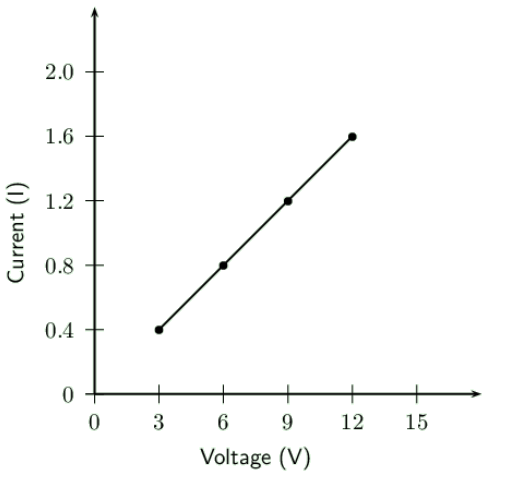What type of graph do you obtain (straight-line, parabola, other curve)

straight-line

Calculate the gradient of the graph.

The gradient of the graph ($$m$$) is the change in the current divided by the change in the voltage:

\begin{align*} m & = \frac{\Delta I}{\Delta V} \\ & = \frac{(\text{1,6}) - (\text{0,4})}{(\text{12}) - (\text{3})} \\ & = \text{0,13} \end{align*}

Do your experimental results verify Ohm's Law? Explain.

Yes. A straight line graph is obtained when we plot a graph of voltage vs. current.

How would you go about finding the resistance of an unknown resistor using only a power supply, a voltmeter and a known resistor $$R_{0}$$?

You start by connecting the known resistor in a circuit with the power supply. Now you read the voltage of the power supply and note this down.

Next you connect the two resistors in series. You can now take the voltage measurements for each of resistors.

So we can find the voltages for the two resistors. Now we note that:

$V = IR$

So using this and the fact that for resistors in series, the current is the same everywhere in the circuit we can find the unknown resistance.

\begin{align*} V_{0} & = IR_{0} \\ I & = \frac{V_{0}}{R_{0}} \\ V_{U} & = IR_{U} \\ I & = \frac{V_{U}}{R_{U}} \\ \frac{V_{U}}{R_{U}} & = \frac{V_{0}}{R_{0}} \\ \therefore R_{U} & = \frac{V_{U}R_{0}}{V_{0}} \end{align*}

### Ohmic and non-ohmic conductors (ESBQ7)

Conductors which obey Ohm's Law have a constant resistance when the voltage is varied across them or the current through them is increased. These conductors are called ohmic conductors. A graph of the current vs. the voltage across these conductors will be a straight-line. Some examples of ohmic conductors are circuit resistors and nichrome wire.

As you have seen, there is a mention of constant temperature when we talk about Ohm's Law. This is because the resistance of some conductors changes as their temperature changes. These types of conductors are called non-ohmic conductors, because they do not obey Ohm's Law. A light bulb is a common example of a non-ohmic conductor. Other examples of non-ohmic conductors are diodes and transistors.

In a light bulb, the resistance of the filament wire will increase dramatically as it warms from room temperature to operating temperature. If we increase the supply voltage in a real lamp circuit, the resulting increase in current causes the filament to increase in temperature, which increases its resistance. This effectively limits the increase in current. In this case, voltage and current do not obey Ohm's Law.

The phenomenon of resistance changing with variations in temperature is one shared by almost all metals, of which most wires are made. For most applications, these changes in resistance are small enough to be ignored. In the application of metal lamp filaments, which increase a lot in temperature (up to about $$\text{1 000}$$ $$\text{℃}$$, and starting from room temperature) the change is quite large.

In general, for non-ohmic conductors, a graph of voltage against current will not be a straight-line, indicating that the resistance is not constant over all values of voltage and current.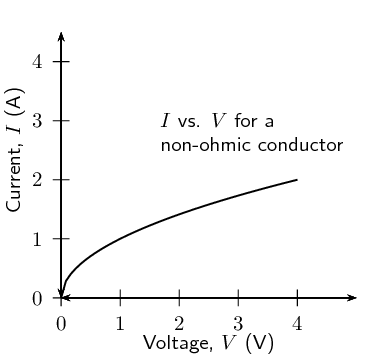A recommended experiment for informal assessment is included. In this experiment learners will obtain current and voltage data for a resistor and light bulb and determine which obeys Ohm's law. You will need light bulbs, resistors, connecting wires, power source, ammeter and voltmeter. Learners should find that the resistor obeys Ohm's law, while the light bulb does not.

## Ohmic and non-ohmic conductors

### Aim

To determine whether two circuit elements (a resistor and a lightbulb) obey Ohm's Law

### Apparatus

4 cells, a resistor, a lightbulb, connecting wires, a voltmeter, an ammeter### Method

The two circuits shown in the diagrams above are the same, except in the first there is a resistor and in the second there is a lightbulb. Set up both the circuits above, starting with 1 cell. For each circuit:

1. Measure the voltage across the circuit element (either the resistor or lightbulb) using the voltmeter.

2. Measure the current in the circuit using the ammeter.

### Results

Draw two tables which look like the following in your book. You should have one table for the first circuit measurements with the resistor and another table for the second circuit measurements with the lightbulb.

 Number of cells Voltage, V ($$\text{V}$$) Current, I ($$\text{A}$$) $$\text{1}$$ $$\text{2}$$ $$\text{3}$$ $$\text{4}$$

### Analysis

Using the data in your tables, draw two graphs of $$I$$ ($$y$$-axis) vs. $$V$$ ($$x$$-axis), one for the resistor and one for the lightbulb.

### Questions and Discussion

1. What should the graph of $$I$$ vs. $$V$$ look like for a conductor which obeys Ohm's Law?

2. Do either or both your graphs look like this?

3. What can you conclude about whether or not the resistor and/or the lightbulb obey Ohm's Law?

4. Is the lightbulb an ohmic or non-ohmic conductor?

### Using Ohm's Law (ESBQ8)

We are now ready to see how Ohm's Law is used to analyse circuits.

Consider a circuit with a cell and an ohmic resistor, R. If the resistor has a resistance of $$\text{5}$$ $$\text{Ω}$$ and voltage across the resistor is $$\text{5}$$ $$\text{V}$$, then we can use Ohm's Law to calculate the current flowing through the resistor. Our first task is to draw the circuit diagram. When solving any problem with electric circuits it is very important to make a diagram of the circuit before doing any calculations. The circuit diagram for this problem looks like the following: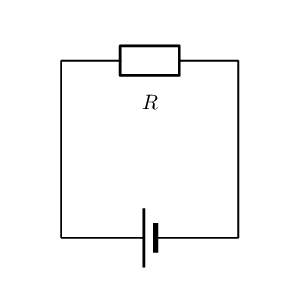The equation for Ohm's Law is: $R = \frac{V}{I}$

which can be rearranged to: $I = \frac{V}{R}$

The current flowing through the resistor is:

\begin{align*} I &= \frac{V}{R} \\ &= \frac{\text{5}\text{ V}}{\text{5 }\Omega} \\ &= \text{1}\text{ A} \end{align*}

temp text

## Worked example 1: Ohm's Law

Study the circuit diagram below: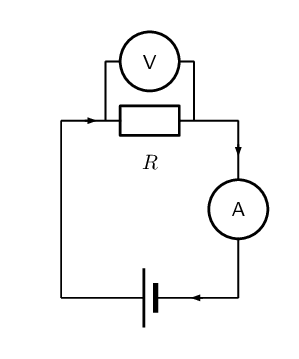The resistance of the resistor is $$\text{10}$$ $$\text{Ω}$$ and the current going through the resistor is $$\text{4}$$ $$\text{A}$$. What is the potential difference (voltage) across the resistor?

### Determine how to approach the problem

We are given the resistance of the resistor and the current passing through it and are asked to calculate the voltage across it. We can apply Ohm's Law to this problem using: $R = \frac{V}{I}.$

### Solve the problem

Rearrange the equation above and substitute the known values for $$R$$ and $$I$$ to solve for $$V$$. \begin{align*} R &= \frac{V}{I} \\ R \times I&= \frac{V}{I} \times I\\ V &= I \times R \\ &= \text{10} \times \text{4} \\ &= \text{40}\text{ V} \end{align*}

The voltage across the resistor is $$\text{40}$$ $$\text{V}$$.

## Ohm's Law

Textbook Exercise 11.2

Calculate the resistance of a resistor that has a potential difference of $$\text{8}$$ $$\text{V}$$ across it when a current of $$\text{2}$$ $$\text{A}$$ flows through it. Draw the circuit diagram before doing the calculation.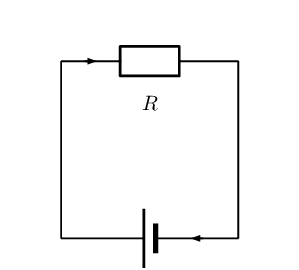The resistance of the unknown resistor is:

\begin{align*} R & = \frac{V}{I} \\ & = \frac{8}{2} \\ & = \text{4}\text{ Ω} \end{align*}

What current will flow through a resistor of $$\text{6}$$ $$\text{Ω}$$ when there is a potential difference of $$\text{18}$$ $$\text{V}$$ across its ends? Draw the circuit diagram before doing the calculation.The resistance of the unknown resistor is:

\begin{align*} I & = \frac{V}{R} \\ & = \frac{18}{6} \\ & = \text{3}\text{ A} \end{align*}

What is the voltage across a $$\text{10}$$ $$\text{Ω}$$ resistor when a current of $$\text{1,5}$$ $$\text{A}$$ flows though it? Draw the circuit diagram before doing the calculation.The resistance of the unknown resistor is:

\begin{align*} V & = I \cdot R \\ & = (\text{1,5})(10) \\ & = \text{15}\text{ V} \end{align*}

### Recap of resistors in series and parallel (ESBQ9)

In Grade 10, you learnt about resistors and were introduced to circuits where resistors were connected in series and in parallel. In a series circuit there is one path along which current flows. In a parallel circuit there are multiple paths along which current flows.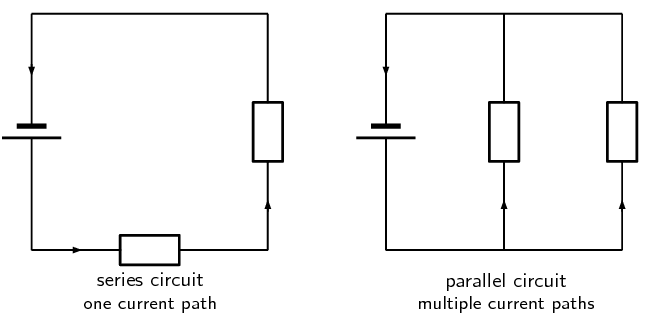When there is more than one resistor in a circuit, we are usually able to calculate the total combined resistance of all the resistors. This is known as the equivalent resistance.

#### Equivalent series resistance

In a circuit where the resistors are connected in series, the equivalent resistance is just the sum of the resistances of all the resistors.

Equivalent resistance in a series circuit,

For n resistors in series the equivalent resistance is:

$R_{s} = R_{1} + R_{2} + R_{3} + \ldots + R_{n}$

Let us apply this to the following circuit.The resistors are in series, therefore:

\begin{align*} R_{s} & = R_{1} + R_{2} + R_{3} \\ & = \text{3}\text{ Ω} + \text{10}\text{ Ω} + \text{5}\text{ Ω} \\ & =\text{18}\text{ Ω} \end{align*}

#### Equivalent parallel resistance

In a circuit where the resistors are connected in parallel, the equivalent resistance is given by the following definition.

Equivalent resistance in a parallel circuit

For $$n$$ resistors in parallel, the equivalent resistance is:

$\frac{1}{R_{p}} = \frac{1}{R_{1}} + \frac{1}{R_{2}} + \frac{1}{R_{3}} + \ldots + \frac{1}{R_{n}}$

Let us apply this formula to the following circuit.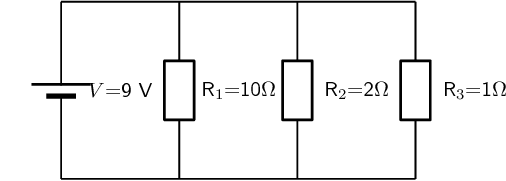What is the total (equivalent) resistance in the circuit?

\begin{align*} \frac{1}{R_{p}} & = \left( \frac{1}{R_{1}} + \frac{1}{R_{2}} + \frac{1}{R_{3}} \right) \\ & = \left( \frac{1}{\text{10}\text{ Ω}} + \frac{1}{\text{2}\text{ Ω}} + \frac{1}{\text{1}\text{ Ω}} \right) \\ & = \left( \frac{\text{1}\text{ Ω} + \text{5}\text{ Ω} + \text{10}\text{ Ω}}{\text{10}\text{ Ω}} \right) \\ & = \left( \frac{\text{16}\text{ Ω}}{\text{10}\text{ Ω}} \right) \\ R_{p} & = \text{0,625}\text{ Ω} \end{align*}

## Series and parallel resistance

Textbook Exercise 11.3

Two $$\text{10}$$ $$\text{kΩ}$$ resistors are connected in series. Calculate the equivalent resistance.

Since the resistors are in series we can use:

$R_{s} = R_{1} + R_{2}$

The equivalent resistance is:

\begin{align*} R_{s} & = R_{1} + R_{2} \\ & = \text{10}\text{ kΩ} + \text{10}\text{ kΩ} \\ & = \text{20}\text{ kΩ} \end{align*}

Two resistors are connected in series. The equivalent resistance is $$\text{100}$$ $$\text{Ω}$$. If one resistor is $$\text{10}$$ $$\text{Ω}$$, calculate the value of the second resistor.

Since the resistors are in series we can use:

$R_{s} = R_{1} + R_{2}$

The equivalent resistance is:

\begin{align*} R_{s} & = R_{1} + R_{2} \\ R_{2} & = R_{s} - R_{1} \\ & = \text{100}\text{ Ω} - \text{10}\text{ Ω} \\ & = \text{90}\text{ Ω} \end{align*}

Two $$\text{10}$$ $$\text{kΩ}$$ resistors are connected in parallel. Calculate the equivalent resistance.

Since the resistors are in parallel we can use:

$\frac{1}{R_{p}} = \frac{1}{R_{1}} + \frac{1}{R_{2}}$

The equivalent resistance is:

\begin{align*} \frac{1}{R_{p}} & = \frac{1}{R_{1}} + \frac{1}{R_{2}} \\ & = \frac{1}{\text{100}} + \frac{1}{\text{10}} \\ & = \frac{1 + 10}{\text{100}} \\ & = \frac{11}{\text{100}} \\ R_{p} & = \text{9,09}\text{ kΩ} \end{align*}

Two resistors are connected in parallel. The equivalent resistance is $$\text{3,75}$$ $$\text{Ω}$$. If one resistor has a resistance of $$\text{10}$$ $$\text{Ω}$$, what is the resistance of the second resistor?

Since the resistors are in parallel we can use:

$\frac{1}{R_{p}} = \frac{1}{R_{1}} + \frac{1}{R_{2}}$

The equivalent resistance is:

\begin{align*} \frac{1}{R_{p}} & = \frac{1}{R_{1}} + \frac{1}{R_{2}} \\ \frac{1}{R_{2}} & = \frac{1}{R_{p}} - \frac{1}{R_{1}} \\ & = \frac{1}{\text{3,75}} - \frac{1}{\text{10}} \\ & = \frac{\text{10} - \text{3,75}}{\text{37,5}} \\ & = \frac{\text{6,25}}{\text{37,5}} \\ R_{2} & = \text{6}\text{ Ω} \end{align*}

Calculate the equivalent resistance in each of the following circuits: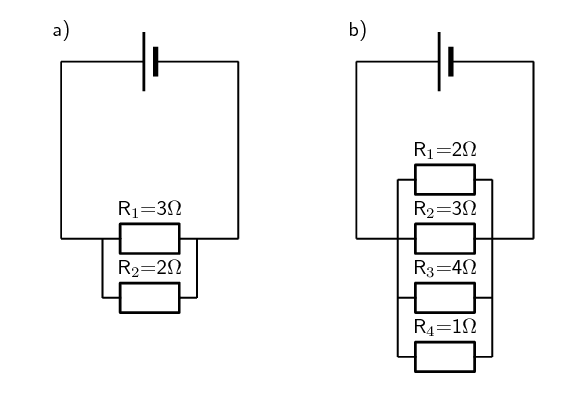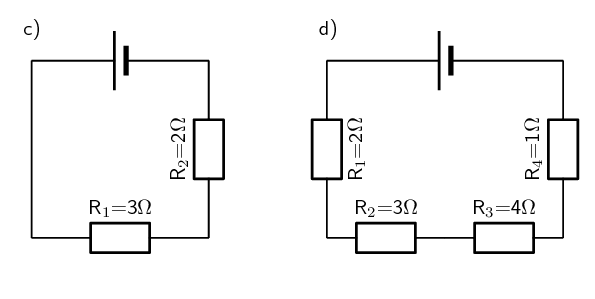a) The resistors are in parallel and so we use:

$\frac{1}{R_{p}} = \frac{1}{R_{1}} + \frac{1}{R_{2}}$

The equivalent resistance is:

\begin{align*} \frac{1}{R_{p}} & = \frac{1}{R_{1}} + \frac{1}{R_{2}} \\ & = \frac{1}{\text{3}} + \frac{1}{\text{2}} \\ & = \frac{\text{2} + \text{3}}{\text{6}} \\ & = \frac{\text{5}}{\text{6}} \\ R & = \text{1,2}\text{ Ω} \end{align*}

b) The resistors are in parallel and so we use:

$\frac{1}{R_{p}} = \frac{1}{R_{1}} + \frac{1}{R_{2}} + \frac{1}{R_{3}} + \frac{1}{R_{4}}$

The equivalent resistance is:

\begin{align*} \frac{1}{R_{p}} & = \frac{1}{R_{1}} + \frac{1}{R_{2}} + \frac{1}{R_{3}} + \frac{1}{R_{4}} \\ & = \frac{1}{\text{2}} + \frac{1}{\text{3}} + \frac{1}{\text{4}} + \frac{1}{\text{1}} \\ & = \frac{\text{6} + \text{4} + \text{3} + \text{12}}{\text{12}} \\ & = \frac{\text{25}}{\text{12}} \\ R & = \text{0,48}\text{ Ω} \end{align*}

c) The resistors are in series and so we use:

$R_{s} = R_{1} + R_{2}$

The equivalent resistance is:

\begin{align*} R_{s} & = R_{1} + R_{2} \\ & = \text{2}\text{ Ω} + \text{3}\text{ Ω} \\ & = \text{5}\text{ Ω} \end{align*}

d) The resistors are in series and so we use:

$R_{s} = R_{1} + R_{2} + R_{3} + R_{4}$

The equivalent resistance is:

\begin{align*} R_{s} & = R_{1} + R_{2} + R_{3} + R_{4} \\ & = \text{2}\text{ Ω} + \text{3}\text{ Ω} + \text{4}\text{ Ω} + \text{1}\text{ Ω} \\ & = \text{10}\text{ Ω} \end{align*}

### Use of Ohm's Law in series and parallel circuits (ESBQB)

Using the definitions for equivalent resistance for resistors in series or in parallel, we can analyse some circuits with these setups.

#### Series circuits

Consider a circuit consisting of three resistors and a single cell connected in series.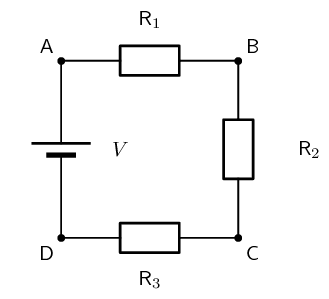The first principle to understand about series circuits is that the amount of current is the same through any component in the circuit. This is because there is only one path for electrons to flow in a series circuit. From the way that the battery is connected, we can tell in which direction the current will flow. We know that current flows from positive to negative by convention. Conventional current in this circuit will flow in a clockwise direction, from point A to B to C to D and back to A.

We know that in a series circuit the current has to be the same in all components. So we can write:

$I = I_{1} = I_{2} = I_{3}.$

We also know that total voltage of the circuit has to be equal to the sum of the voltages over all three resistors. So we can write:

$V = V_{1} + V_{2} + V_{3}$

Using this information and what we know about calculating the equivalent resistance of resistors in series, we can approach some circuit problems.

## Worked example 2: Ohm's Law, series circuit

Calculate the current (I) in this circuit if the resistors are both ohmic in nature.### Determine what is required

We are required to calculate the current flowing in the circuit.

### Determine how to approach the problem

Since the resistors are ohmic in nature, we can use Ohm's Law. There are however two resistors in the circuit and we need to find the total resistance.

### Find total resistance in circuit

Since the resistors are connected in series, the total (equivalent) resistance R is:

$R = R_{1} + R_{2}$

Therefore,

\begin{align*} R & = \text{2} + \text{4} \\ & = \text{6}\text{ Ω} \end{align*}

### Apply Ohm's Law

\begin{align*} R & = \frac{V}{I} \\ R \times \frac{I}{R} & = \frac{V}{I} \times \frac{I}{R} \\ I & = \frac{V}{R} \\ & = \frac{12}{6} \\ & = \text{2}\text{ A} \end{align*}

A current of $$\text{2}$$ $$\text{A}$$ is flowing in the circuit.

## Worked example 3: Ohm's Law, series circuit

Two ohmic resistors ($$R_{1}$$ and $$R_{2}$$) are connected in series with a cell. Find the resistance of $$R_{2}$$, given that the current flowing through $$R_{1}$$ and $$R_{2}$$ is $$\text{0,25}$$ $$\text{A}$$ and that the voltage across the cell is $$\text{1,5}$$ $$\text{V}$$. $$R_{1}$$ =$$\text{1}$$ $$\text{Ω}$$.

### Draw the circuit and fill in all known values.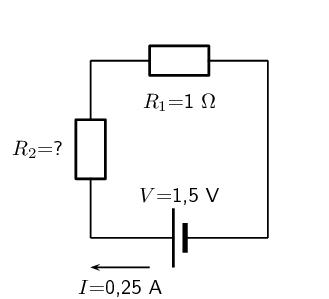### Determine how to approach the problem.

We can use Ohm's Law to find the total resistance R in the circuit, and then calculate the unknown resistance using:

$R = R_{1} + R_{2}$

because it is in a series circuit.

### Find the total resistance

\begin{align*} R & = \frac{V}{I} \\ & = \frac{\text{1,5}}{\text{0,25}} \\ & = \text{6}\text{ Ω} \end{align*}

### Find the unknown resistance

We know that:

$R = \text{6}\text{ Ω}$

and that

$R_{1} = \text{1}\text{ Ω}$

Since

$R = R_{1} + R_{2}$ $R_{2} = R - R_{1}$

Therefore,

$R_{1} = \text{5}\text{ Ω}$

## Worked example 4: Ohm's Law, series circuit

For the following circuit, calculate:

1. the voltage drops $$V_1$$, $$V_2$$ and $$V_3$$ across the resistors $$R_1$$, $$R_2$$, and $$R_3$$

2. the resistance of $$R_3$$.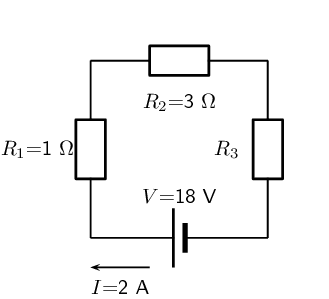### Determine how to approach the problem

We are given the voltage across the cell and the current in the circuit, as well as the resistances of two of the three resistors. We can use Ohm's Law to calculate the voltage drop across the known resistors. Since the resistors are in a series circuit the voltage is $$V = V_1 + V_2 + V_3$$ and we can calculate $$V_3$$. Now we can use this information to find the voltage across the unknown resistor $$R_3$$.

### Calculate voltage drop across $$R_1$$

Using Ohm's Law: \begin{align*} R_1 &= \frac{V_1}{I} \\ I \cdot R_1 &= I \cdot \frac{V_1}{I} \\ V_1 &= {I}\cdot{R_1}\\ &= 2 \cdot 1 \\ V_1 &= \text{2}\text{ V} \end{align*}

### Calculate voltage drop across $$R_2$$

Again using Ohm's Law: \begin{align*} R_2 &= \frac{V_2}{I} \\ I \cdot R_2 &= I \cdot \frac{V_2}{I} \\ V_2 &= {I}\cdot{R_2}\\ &= 2 \cdot 3 \\ V_2 &= \text{6}\text{ V} \end{align*}

### Calculate voltage drop across $$R_3$$

Since the voltage drop across all the resistors combined must be the same as the voltage drop across the cell in a series circuit, we can find $$V_3$$ using: \begin{align*} V &= V_1 + V_2 + V_3\\ V_3 &= V - V_1 - V_2 \\ &= 18 - 2 - 6 \\ V_3&= \text{10}\text{ V} \end{align*}

### Find the resistance of $$R_3$$

We know the voltage across $$R_3$$ and the current through it, so we can use Ohm's Law to calculate the value for the resistance: \begin{align*} R_3 &= \frac{V_3}{I}\\ &= \frac{10}{2} \\ R_3&= \text{5 } \Omega \end{align*}

$$V_1 = \text{2}\text{ V}$$

$$V_2 = \text{6}\text{ V}$$

$$V_3 = \text{10}\text{ V}$$

$$R_1 = \text{5 } \Omega$$

temp text

#### Parallel circuits

Consider a circuit consisting of a single cell and three resistors that are connected in parallel.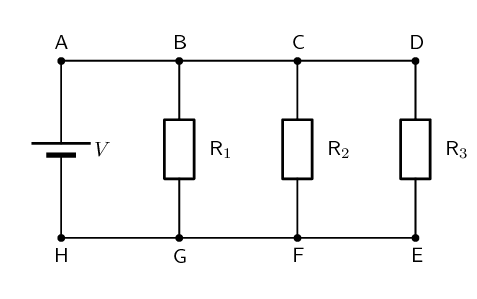The first principle to understand about parallel circuits is that the voltage is equal across all components in the circuit. This is because there are only two sets of electrically common points in a parallel circuit, and voltage measured between sets of common points must always be the same at any given time. So, for the circuit shown, the following is true:

$V = V_{1} = V_{2} = V_{3}.$

The second principle for a parallel circuit is that all the currents through each resistor must add up to the total current in the circuit:

$I = I_{1} + I_{2} + I_{3}.$

Using these principles and our knowledge of how to calculate the equivalent resistance of parallel resistors, we can now approach some circuit problems involving parallel resistors.

## Worked example 5: Ohm's Law, parallel circuit

Calculate the current (I) in this circuit if the resistors are both ohmic in nature.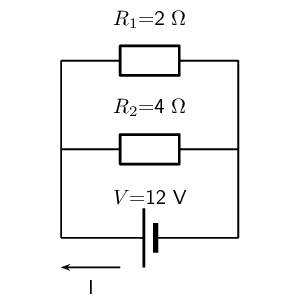### Determine what is required

We are required to calculate the current flowing in the circuit.

### Determine how to approach the problem

Since the resistors are ohmic in nature, we can use Ohm's Law. There are however two resistors in the circuit and we need to find the total resistance.

### Find the equivalent resistance in circuit

Since the resistors are connected in parallel, the total (equivalent) resistance R is:

$\frac{1}{R} = \frac{1}{R_{1}} + \frac{1}{R_{2}}.$ \begin{align*} \frac{1}{R} &= \frac{1}{R_1} + \frac{1}{R_2} \\ &= \frac{1}{2} + \frac{1}{4} \\ &= \frac{2+1}{4} \\ &= \frac{3}{4} \\ \text{Therefore, } R &= \text{1,33 } \Omega \end{align*}

### Apply Ohm's Law

\begin{align*} R&= \frac{V}{I} \\ R \cdot \frac{I}{R} &= \frac{V}{I} \cdot \frac{I}{R} \\ I &= \frac{V}{R}\\ I &= V \cdot \frac{1}{R}\\ &= (12) \left(\frac{3}{4}\right) \\ &= \text{9}\text{ A} \end{align*}

The current flowing in the circuit is $$\text{9}$$ $$\text{A}$$.

## Worked example 6: Ohm's Law, parallel circuit

Two ohmic resistors ($$R_1$$ and $$R_2$$) are connected in parallel with a cell. Find the resistance of $$R_2$$, given that the current flowing through the cell is $$\text{4,8}$$ $$\text{A}$$ and that the voltage across the cell is $$\text{9}$$ $$\text{V}$$.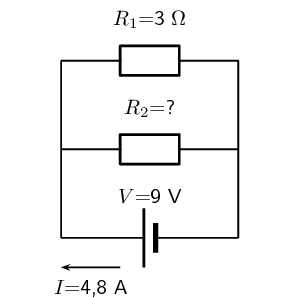### Determine what is required

We need to calculate the resistance $$R_2$$.

### Determine how to approach the problem

Since the resistors are ohmic and we are given the voltage across the cell and the current through the cell, we can use Ohm's Law to find the equivalent resistance in the circuit. \begin{align*} R & = \frac{V}{I} \\ & = \frac{9}{\text{4,8}} \\ & = \text{1,875} \ \Omega \end{align*}

### Calculate the value for $$R_2$$

Since we know the equivalent resistance and the resistance of $$R_1$$, we can use the formula for resistors in parallel to find the resistance of $$R_2$$. \begin{align*} \frac{1}{R} & = \frac{1}{R_1} + \frac{1}{R_2} \end{align*} Rearranging to solve for $$R_2$$: \begin{align*} \frac{1}{R_2} & = \frac{1}{R} - \frac{1}{R_1} \\ & = \frac{1}{\text{1,875}} - \frac{1}{3}\\ & = \text{0,2} \\ R_2 & = \frac{1}{\text{0,2}} \\ & = \text{5} \ \Omega \end{align*}

The resistance $$R_2$$ is $$\text{5}$$ $$\Omega$$

temp text

## Worked example 7: Ohm's Law, parallel circuit

An 18 volt cell is connected to two parallel resistors of $$\text{4}$$ $$\Omega$$ and $$\text{12}$$ $$\Omega$$ respectively. Calculate the current through the cell and through each of the resistors.

### First draw the circuit before doing any calculations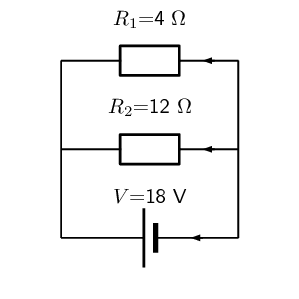### Determine how to approach the problem

We need to determine the current through the cell and each of the parallel resistors. We have been given the potential difference across the cell and the resistances of the resistors, so we can use Ohm's Law to calculate the current.

### Calculate the current through the cell

To calculate the current through the cell we first need to determine the equivalent resistance of the rest of the circuit. The resistors are in parallel and therefore: \begin{align*} \frac{1}{R} &= \frac{1}{R_1} + \frac{1}{R_2} \\ &= \frac{1}{4} + \frac{1}{12} \\ &= \frac{3+1}{12} \\ &= \frac{4}{12} \\ R &= \frac{12}{4} = \text{3} \ \Omega \end{align*} Now using Ohm's Law to find the current through the cell: \begin{align*} R &= \frac{V}{I} \\ I &= \frac{V}{R} \\ &= \frac{18}{3} \\ I &= \text{6}\text{ A} \end{align*}

### Now determine the current through one of the parallel resistors

We know that for a purely parallel circuit, the voltage across the cell is the same as the voltage across each of the parallel resistors. For this circuit: \begin{align*} V &= V_1 = V_2 = \text{18}\text{ V} \end{align*} Let's start with calculating the current through $$R_1$$ using Ohm's Law: \begin{align*} R_1 &= \frac{V_1}{I_1} \\ I_1 &= \frac{V_1}{R_1} \\ &= \frac{18}{4} \\ I_1 &= \text{4,5}\text{ A} \end{align*}

### Calculate the current through the other parallel resistor

We can use Ohm's Law again to find the current in $$R_2$$: \begin{align*} R_2 &= \frac{V_2}{I_2} \\ I_2 &= \frac{V_2}{R_2} \\ &= \frac{18}{12} \\ I_2 &= \text{1,5}\text{ A} \end{align*} An alternative method of calculating $$I_2$$ would have been to use the fact that the currents through each of the parallel resistors must add up to the total current through the cell: \begin{align*} I &= I_1 + I_2 \\ I_2 &= I - I_1 \\ &= 6 - 4.5 \\ I_2 &= \text{1,5}\text{ A} \end{align*}

The current through the cell is $$\text{6}$$ $$\text{A}$$.

The current through the $$\text{4}$$ $$\Omega$$ resistor is $$\text{4,5}$$ $$\text{A}$$.

The current through the $$\text{12}$$ $$\Omega$$ resistor is $$\text{1,5}$$ $$\text{A}$$.

## Ohm's Law in series and parallel circuits

Textbook Exercise 11.4

Calculate the value of the unknown resistor in the circuit: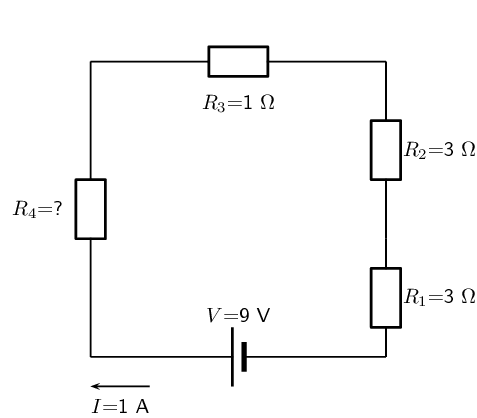We first use Ohm's law to calculate the total series resistance:

\begin{align*} R & = \frac{V}{I} \\ & = \frac{9}{1} \\ & = \text{9}\text{ Ω} \end{align*}

Now we can find the unknown resistance:

\begin{align*} R_{s} & = R_{1} + R_{2} + R_{3} + R_{4} \\ R_{4} & = R_{s} - R_{1} - R_{2} - R_{3} \\ & = 9 - 3 - 3 - 1 \\ & = \text{2}\text{ Ω} \end{align*}

Calculate the value of the current in the following circuit:We first find the total resistance:

\begin{align*} R_{s} & = R_{1} + R_{2} + R_{3} \\ & = \text{1} + \text{2,5} + \text{1,5} \\ & = \text{5}\text{ Ω} \end{align*}

Now we can calculate the current:

\begin{align*} I & = \frac{V}{R} \\ & = \frac{9}{5} \\ & = \text{1,8}\text{ A} \end{align*}

Three resistors with resistance $$\text{1}$$ $$\text{Ω}$$, $$\text{5}$$ $$\text{Ω}$$ and $$\text{10}$$ $$\text{Ω}$$ respectively, are connected in series with a $$\text{12}$$ $$\text{V}$$ battery. Calculate the value of the current in the circuit.

We draw the circuit diagram:We now find the total resistance:

\begin{align*} R_{s} & = R_{1} + R_{2} + R_{3} \\ & = \text{1} + \text{5} + \text{10} \\ & = \text{16}\text{ Ω} \end{align*}

Now we can calculate the current:

\begin{align*} I & = \frac{V}{R} \\ & = \frac{12}{16} \\ & = \text{0,75}\text{ A} \end{align*}

Calculate the current through the cell if the resistors are both ohmic in nature.We first find the total resistance:

\begin{align*} \frac{1}{R_{p}} & = \frac{1}{R_{1}} + \frac{1}{R_{2}} \\ & = \frac{1}{\text{1}} + \frac{1}{\text{3}} \\ & = \frac{3 + 1}{\text{3}} \\ & = \frac{4}{\text{3}} \\ & = \text{0,75}\text{ Ω} \end{align*}

Now we can calculate the current:

\begin{align*} I & = \frac{V}{R} \\ & = \frac{9}{\text{0,75}} \\ & = \text{12}\text{ A} \end{align*}

Calculate the value of the unknown resistor $$R_{4}$$ in the circuit:We first find the total resistance:

\begin{align*} R & = \frac{V}{I} \\ & = \frac{24}{\text{2}} \\ & = \text{12}\text{ Ω} \end{align*}

Now we can calculate the unknown resistance:

\begin{align*} \frac{1}{R_{p}} & = \frac{1}{R_{1}} + \frac{1}{R_{2}} + \frac{1}{R_{3}} + \frac{1}{R_{4}}\\ \frac{1}{R_{4}} & = \frac{1}{R_{p}} - \frac{1}{R_{1}} - \frac{1}{R_{2}} - \frac{1}{R_{3}}\\ & = \frac{1}{\text{12}} - \frac{1}{\text{120}} - \frac{1}{\text{40}} - \frac{1}{\text{60}} \\ & = \frac{10 - 1 - 3 - 2}{\text{120}} \\ & = \frac{4}{\text{120}} \\ & = \text{30}\text{ Ω} \end{align*}

Three resistors with resistance $$\text{1}$$ $$\text{Ω}$$, $$\text{5}$$ $$\text{Ω}$$ and $$\text{10}$$ $$\text{Ω}$$ respectively, are connected in parallel with a $$\text{20}$$ $$\text{V}$$ battery. All the resistors are ohmic in nature. Calculate:

the value of the current through the battery

We draw a circuit diagram: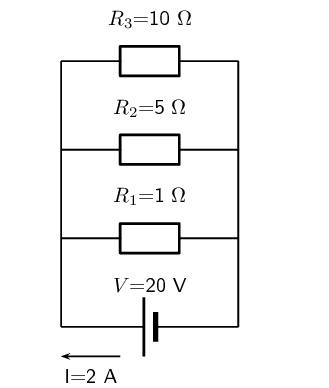To calculate the value of the current through the battery we first need to calculate the equivalent resistance:

\begin{align*} \frac{1}{R_{p}} & = \frac{1}{R_{1}} + \frac{1}{R_{2}} + \frac{1}{R_{3}}\\ & = \frac{1}{\text{1}} + \frac{1}{\text{5}} + \frac{1}{\text{10}} \\ & = \frac{10 + 2 + 1}{\text{10}} \\ & = \frac{13}{\text{10}} \\ & = \text{0,77}\text{ Ω} \end{align*}

Now we can calculate the current through the battery:

\begin{align*} I & = \frac{V}{R} \\ & = \frac{20}{\text{0,77}} \\ & = \text{26}\text{ A} \end{align*}

the value of the current in each of the three resistors.

For a parallel circuit the voltage across cell is the same as the voltage across each of the resistors. For this circuit:

$V = V_{1} = V_{2} = V_{3} = \text{20}\text{ V}$

Now we can calculate the current through each resistor. We will start with $$R_{1}$$:

\begin{align*} I & = \frac{V}{R} \\ & = \frac{20}{\text{1}} \\ & = \text{20}\text{ A} \end{align*}

Next we calculate the current through $$R_{2}$$:

\begin{align*} I & = \frac{V}{R} \\ & = \frac{20}{\text{5}} \\ & = \text{4}\text{ A} \end{align*}

And finally we calculate the current through $$R_{3}$$:

\begin{align*} I & = \frac{V}{R} \\ & = \frac{20}{\text{10}} \\ & = \text{2}\text{ A} \end{align*}

You can check that these add up to the total current.

### Series and parallel networks of resistors (ESBQC)

Now that you know how to handle simple series and parallel circuits, you are ready to tackle circuits which combine these two setups such as the following circuit: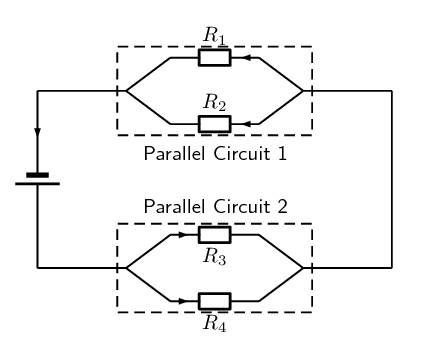Figure 11.1: An example of a series-parallel network. The dashed boxes indicate parallel sections of the circuit.

It is relatively easy to work out these kind of circuits because you use everything you have already learnt about series and parallel circuits. The only difference is that you do it in stages. In Figure 11.1, the circuit consists of 2 parallel portions that are then in series with a cell. To work out the equivalent resistance for the circuit, you start by calculating the total resistance of each of the parallel portions and then add up these resistances in series. If all the resistors in Figure 11.1 had resistances of $$\text{10}$$ $$\text{Ω}$$, we can calculate the equivalent resistance of the entire circuit.

We start by calculating the total resistance of Parallel Circuit 1.The value of $$R_{p1}$$ is: \begin{align*} \frac{1}{R_{p1}} &= \frac{1}{R_1} + \frac{1}{R_2} \\ R_{p1}&= \left(\frac{1}{10} + \frac{1}{10} \right)^{-1} \\ &= \left(\frac{1+1}{10} \right)^{-1} \\ &= \left(\frac{2}{10} \right)^{-1} \\ &= \text{5} \, \Omega \end{align*}

We can similarly calculate the total resistance of Parallel Circuit 2: \begin{align*} \frac{1}{R_{p2}} &= \frac{1}{R_3} + \frac{1}{R_4} \\ R_{p2}&= \left(\frac{1}{10} + \frac{1}{10} \right)^{-1} \\ &= \left(\frac{1+1}{10} \right)^{-1} \\ &= \left(\frac{2}{10} \right)^{-1} \\ &= \text{5} \, \Omega \end{align*}

You can now treat the circuit like a simple series circuit as follows: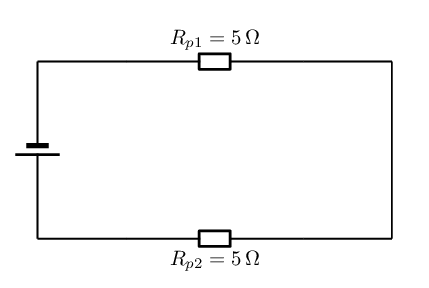Therefore the equivalent resistance is: \begin{align*} R &= R_{p1} + R_{p2} \\ &= 5 + 5 \\ &= 10 \, \Omega \end{align*}

The equivalent resistance of the circuit in Figure 11.1 is $$\text{10}$$ $$\text{Ω}$$.

temp text

## Series and parallel networks

Textbook Exercise 11.5

Determine the equivalent resistance of the following circuits: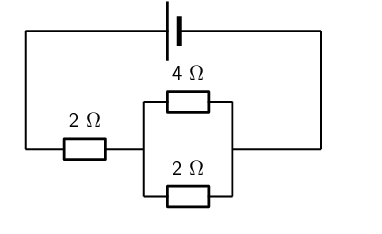We start by determining the equivalent resistance of the parallel combination:

\begin{align*} \frac{1}{R_{p}} & = \frac{1}{R_{1}} + \frac{1}{R_{2}} \\ & = \frac{1}{4} + \frac{1}{2} \\ & = \frac{3}{4}\\ R_{p} & = \text{1,33}\text{ Ω} \end{align*}

Now we have a circuit with two resistors in series so we can calculate the equivalent resistance:

\begin{align*} R_{s} & = R_{3} + R_{p} \\ & = \text{2} + \text{1,33} \\ & = \text{3,33}\text{ Ω} \end{align*}We start by determining the equivalent resistance of the parallel combination:

\begin{align*} \frac{1}{R_{p}} & = \frac{1}{R_{1}} + \frac{1}{R_{2}} \\ & = \frac{1}{1} + \frac{1}{2} \\ & = \frac{3}{2}\\ R_{p} & = \text{0,67}\text{ Ω} \end{align*}

Now we have a circuit with three resistors in series so we can calculate the equivalent resistance:

\begin{align*} R_{s} & = R_{3} + R_{4} + R_{p} \\ & = \text{4} + \text{6} + \text{0,67} \\ & = \text{10,67}\text{ Ω} \end{align*}We start by determining the equivalent resistance of the parallel combination:

\begin{align*} \frac{1}{R_{p}} & = \frac{1}{R_{1}} + \frac{1}{R_{2}} + \frac{1}{R_{3}} \\ & = \frac{1}{3} + \frac{1}{5} + \frac{1}{1} \\ & = \frac{23}{15}\\ R_{p} & = \text{0,652}\text{ Ω} \end{align*}

Now we have a circuit with two resistors in series so we can calculate the equivalent resistance:

\begin{align*} R_{s} & = R_{4} + R_{p} \\ & = \text{2} + \text{0,652} \\ & = \text{2,652}\text{ Ω} \end{align*}

Examine the circuit below: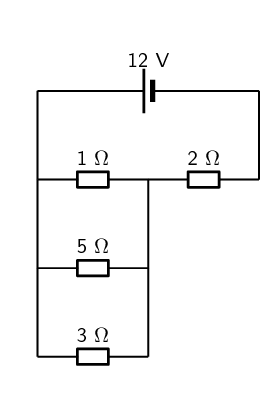If the potential difference across the cell is $$\text{12}$$ $$\text{V}$$, calculate:

the current $$I$$ through the cell.

To find the current $$I$$ we first need to find the equivalent resistance. We start by calculating the equivalent resistance of the parallel combination:

\begin{align*} \frac{1}{R_{p}} & = \frac{1}{R_{1}} + \frac{1}{R_{2}} + \frac{1}{R_{3}} \\ & = \frac{1}{3} + \frac{1}{5} + \frac{1}{1} \\ & = \frac{23}{15}\\ R_{p} & = \text{0,652}\text{ Ω} \end{align*}

Now we have a circuit with two resistors in series so we can calculate the equivalent resistance:

\begin{align*} R_{s} & = R_{4} + R_{p} \\ & = \text{2} + \text{0,652} \\ & = \text{2,652}\text{ Ω} \end{align*}

So the current through the cell is:

\begin{align*} I & = \frac{V}{R} \\ & = \frac{\text{12}}{\text{2,652}} \\ & = \text{4,52}\text{ A} \end{align*}

the current through the $$\text{5}$$ $$\text{Ω}$$ resistor.

The current through the parallel combination of resistors is $$\text{4,52}$$ $$\text{A}$$. (The current is the same through series combinations of resistors and we can consider the entire parallel set of resistors as one series resistor.)

Using this we can find the voltage through the parallel combination of resistors (remember to use the equivalent parallel resistance and not the equivalent resistance of the circuit):

\begin{align*} V & = I \cdot R \\ & = (\text{4,52})(\text{0,652}) \\ & = \text{2,95}\text{ V} \end{align*}

Since the voltage across each resistor in the parallel combination is the same, this is also the voltage across the $$\text{5}$$ $$\text{Ω}$$ resistor.

So now we can calculate the current through the resistor:

\begin{align*} I & = \frac{V}{R} \\ & = \frac{\text{2,95}}{\text{5}} \\ & = \text{0,59}\text{ A} \end{align*}

If current flowing through the cell is $$\text{2}$$ $$\text{A}$$, and all the resistors are ohmic, calculate the voltage across the cell and each of the resistors, $$R_1$$, $$R_2$$, and $$R_3$$ respectively.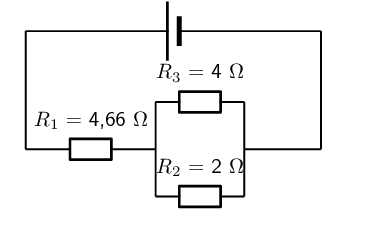To find the voltage we first need to find the equivalent resistance. We start by calculating the equivalent resistance of the parallel combination:

\begin{align*} \frac{1}{R_{p}} & = \frac{1}{R_{2}} + \frac{1}{R_{3}} \\ & = \frac{1}{2} + \frac{1}{4} \\ & = \frac{3}{4}\\ R_{p} & = \text{1,33}\text{ Ω} \end{align*}

Now we have a circuit with two resistors in series so we can calculate the equivalent resistance:

\begin{align*} R_{s} & = R_{1} + R_{p} \\ & = \text{4,66} + \text{1,33} \\ & = \text{5,99}\text{ Ω} \end{align*}

So the voltage across the cell is:

\begin{align*} V & = I \cdot R \\ & = (\text{2})(\text{5,99}) \\ & = \text{12}\text{ V} \end{align*}

The current through the parallel combination of resistors is $$\text{2}$$ $$\text{A}$$. (The current is the same through series combinations of resistors and we can consider the entire parallel set of resistors as one series resistor.)

Using this we can find the voltage through the each of the resistors. We start by finding the voltage across $$R_{1}$$:

\begin{align*} V & = I \cdot R \\ & = (\text{2})(\text{4,66}) \\ & = \text{9,32}\text{ V} \end{align*}

Now we find the voltage across the parallel combination:

\begin{align*} V & = I \cdot R \\ & = (\text{2})(\text{1,33}) \\ & = \text{2,66}\text{ V} \end{align*}

Since the voltage across each resistor in the parallel combination is the same, this is also the voltage across resistors $$R_{2}$$ and $$R_{3}$$.

For the following circuit, calculate: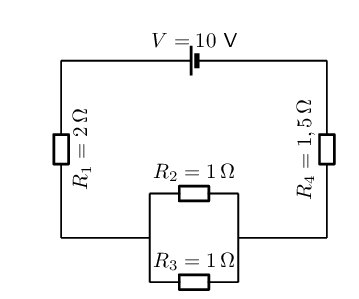the current through the cell

To find the current we first need to find the equivalent resistance. We start by calculating the equivalent resistance of the parallel combination:

\begin{align*} \frac{1}{R_{p}} & = \frac{1}{R_{2}} + \frac{1}{R_{3}} \\ & = \frac{1}{1} + \frac{1}{1} \\ & = 2\\ R_{p} & = \text{0,5}\text{ Ω} \end{align*}

Now we have a circuit with two resistors in series so we can calculate the equivalent resistance:

\begin{align*} R_{s} & = R_{1} + R_{4} + R_{p} \\ & = \text{2} + \text{1,5} + \text{0,5} \\ & = \text{4}\text{ Ω} \end{align*}

So the current through the cell is:

\begin{align*} I & = \frac{V}{R} \\ & = \frac{\text{10}}{\text{4}} \\ & = \text{2,5}\text{ A} \end{align*}

the voltage drop across $$R_4$$

The current through all the resistors is $$\text{2,5}$$ $$\text{A}$$. (The current is the same through series combinations of resistors and we can consider the entire parallel set of resistors as one series resistor.)

Using this we can find the voltage through $$R_{4}$$:

\begin{align*} V & = I \cdot R \\ & = (\text{2,5})(\text{1,5}) \\ & = \text{3,75}\text{ V} \end{align*}

the current through $$R_2$$

The current through all the resistors is $$\text{2,5}$$ $$\text{A}$$. (The current is the same through series combinations of resistors and we can consider the entire parallel set of resistors as one series resistor.)

Using this we can find the current through $$R_{2}$$.

We first need to find the voltage across the parallel combination:

\begin{align*} V & = I \cdot R \\ & = (\text{2,5})(\text{0,5}) \\ & = \text{1,25}\text{ V} \end{align*}

Now we can find the current through $$R_{2}$$ using the fact that the voltage is the same across each resistor in the parallel combination:

\begin{align*} I & = \frac{V}{R} \\ & = \frac{\text{1,25}}{\text{1}} \\ & = \text{1,25}\text{ A} \end{align*}